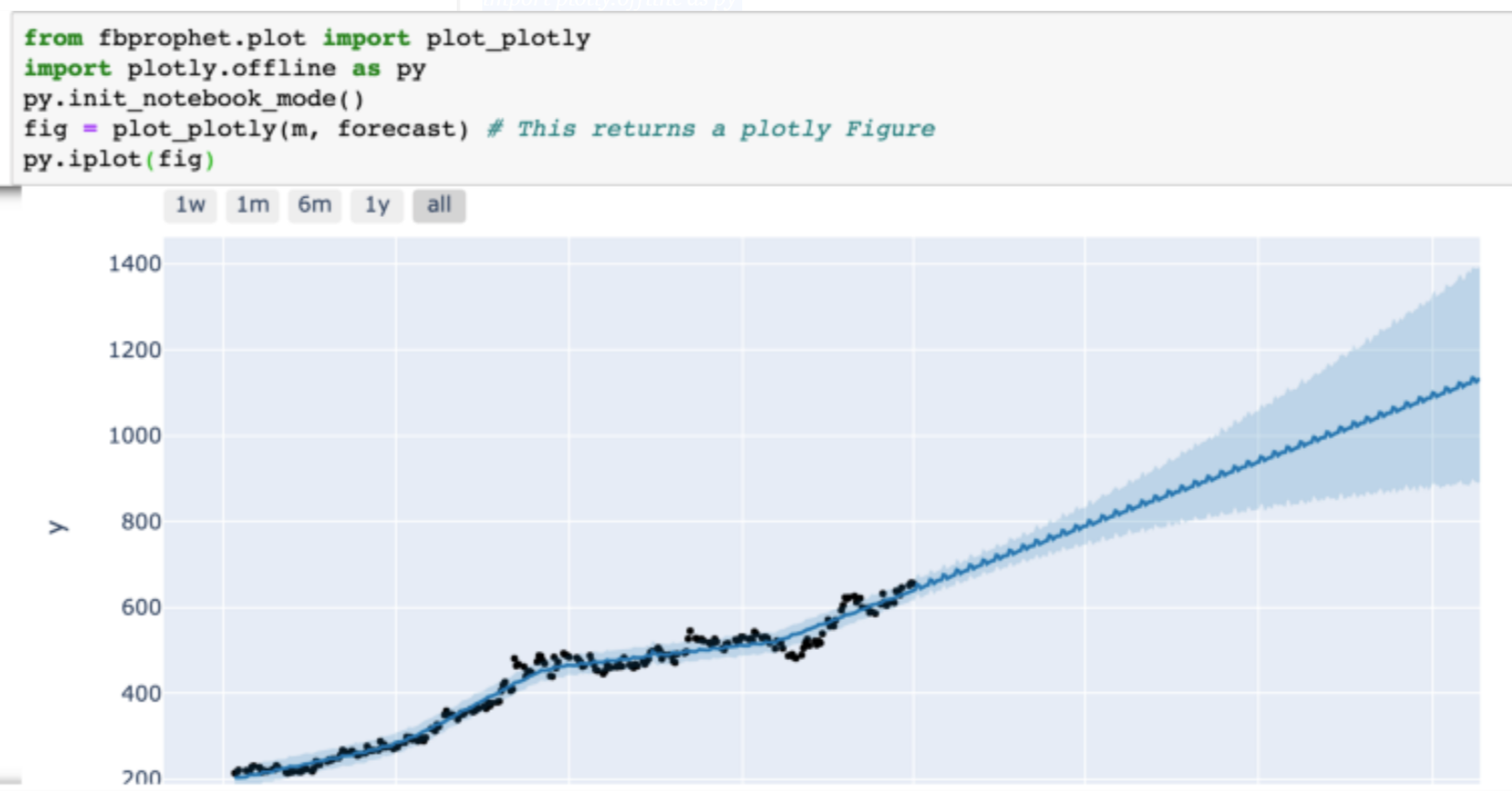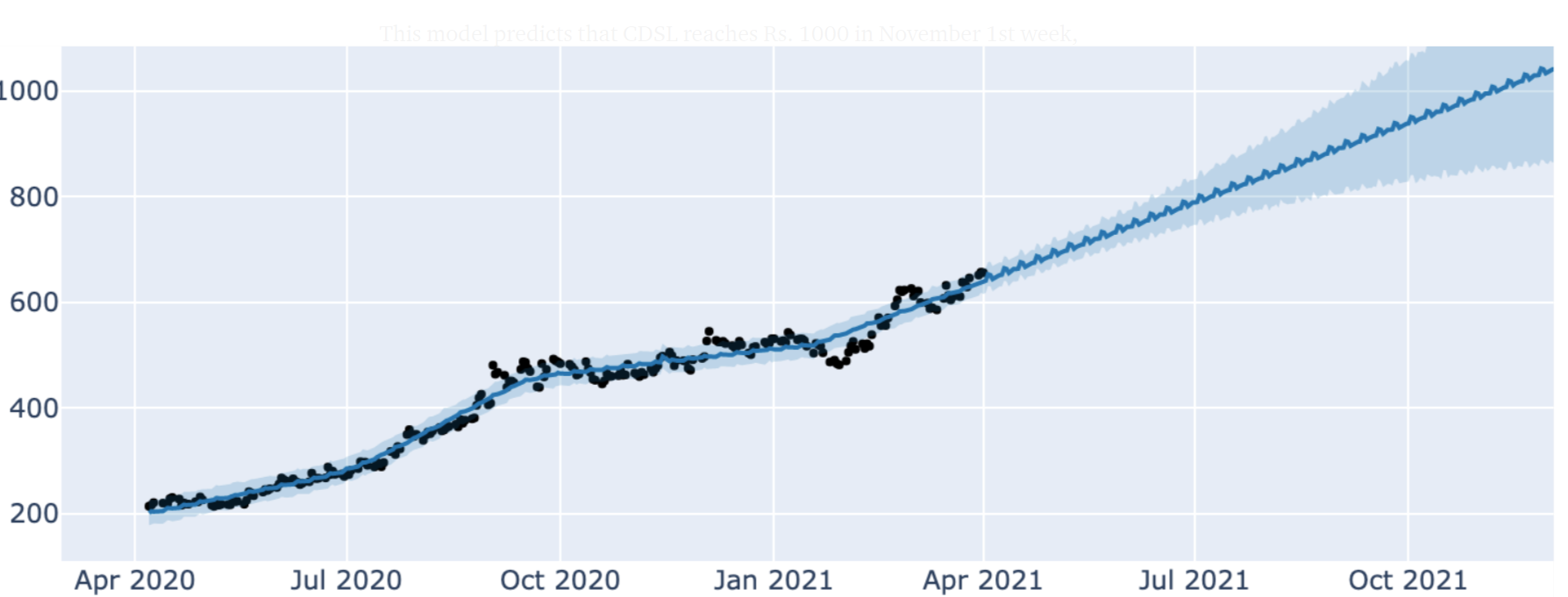# 使用机器学习预测股价

1. 股票价格不是随机的，它们的确遵循过去的历史趋势
2. 人为的决定是情感和理性的结合，倾向于查看过去的数据
3. 市场永远是对的。他们只会惩罚错误的决定
4. 贪婪，盲目打电话，高风险的食欲，谨慎行事可以根据时间奖励或惩罚投资者。

# 预测

``````import pandas as pd
``````

CDSL在下面显示带有标题的数据。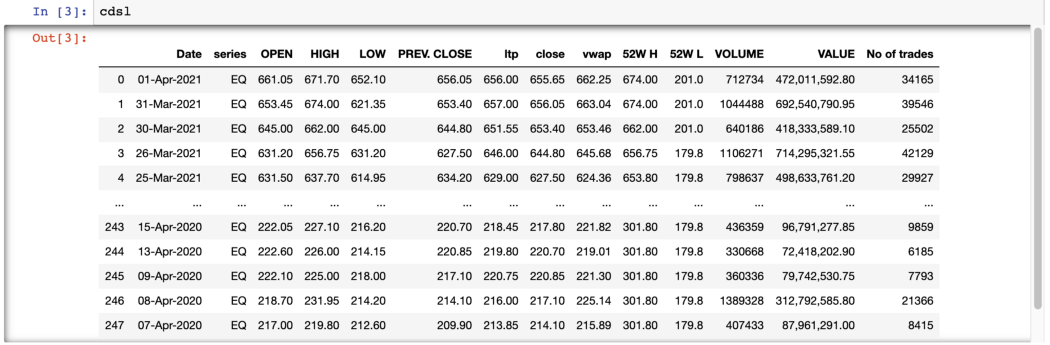``````list(cdsl.columns)
``````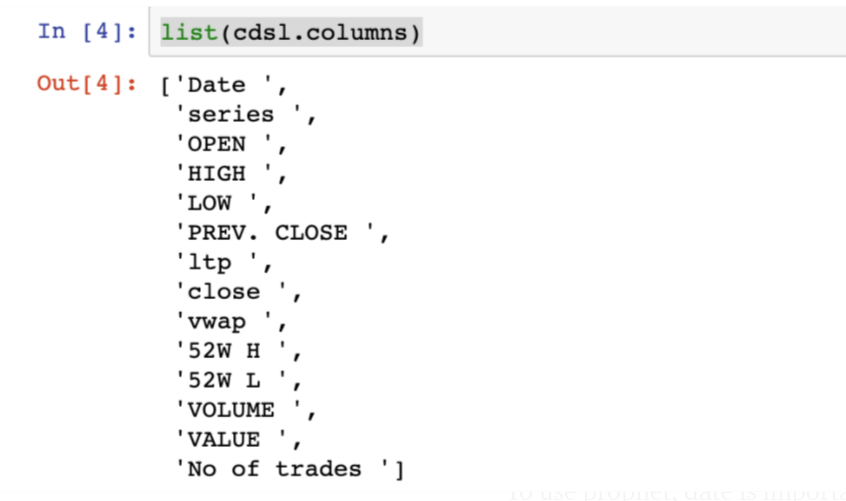``````df = cdsl.rename(columns = {‘Date ‘: ‘Date’, ‘series ‘: ‘series’, ‘OPEN ‘: ‘Open’ , ‘HIGH ‘: ‘High’, ‘LOW ‘: ‘Low’, ‘PREV. CLOSE ‘: ‘PREV. CLOSE’, ‘ltp ‘: ‘ltp’, ‘close ‘: ‘close’, ‘vwap ‘: ‘vwap’, ‘52W H ‘: ‘52W H’, ‘52W L ‘: ‘52W L’ , ‘VOLUME ‘: ‘VOLUME’, ‘No of trades ‘:’No of trades’ }, inplace = False)
``````

``````cdsl ['Date']
``````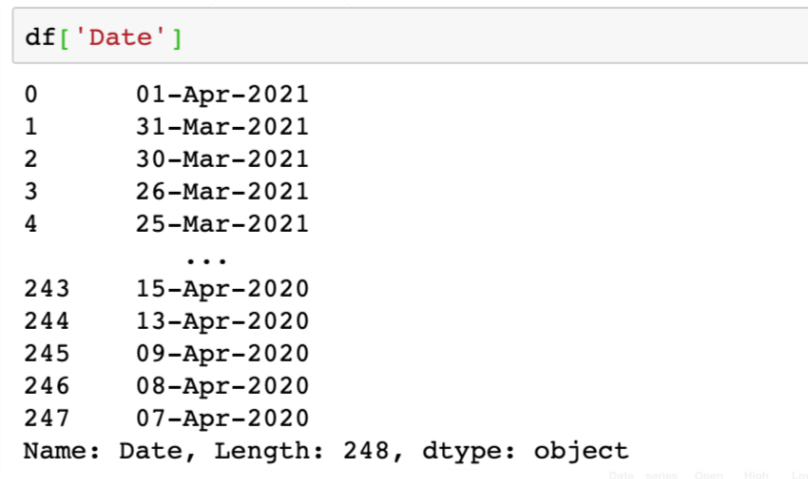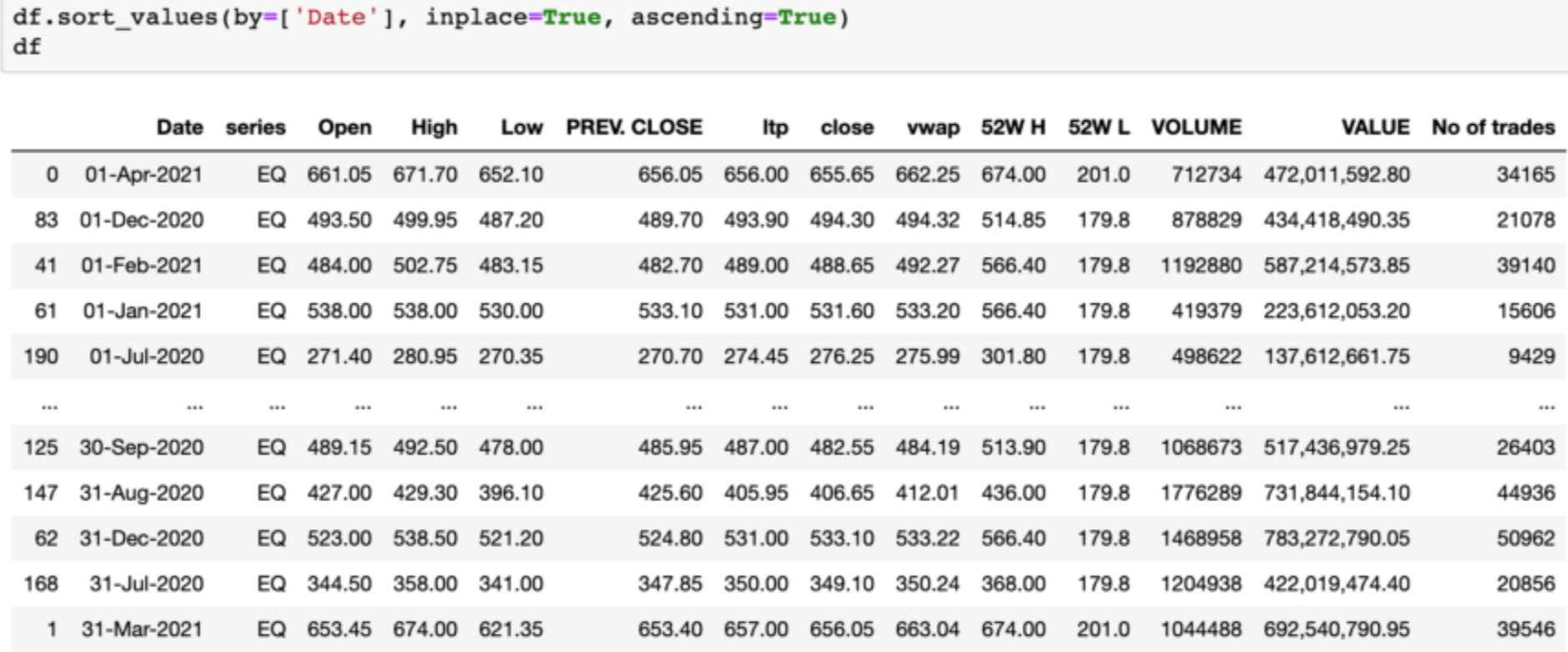``````df[‘Date’] = pd.to_datetime(df.Date,format=’%d-%b-%Y’)
df.sort_values(by=[‘Date’], inplace=True, ascending=True)
df
``````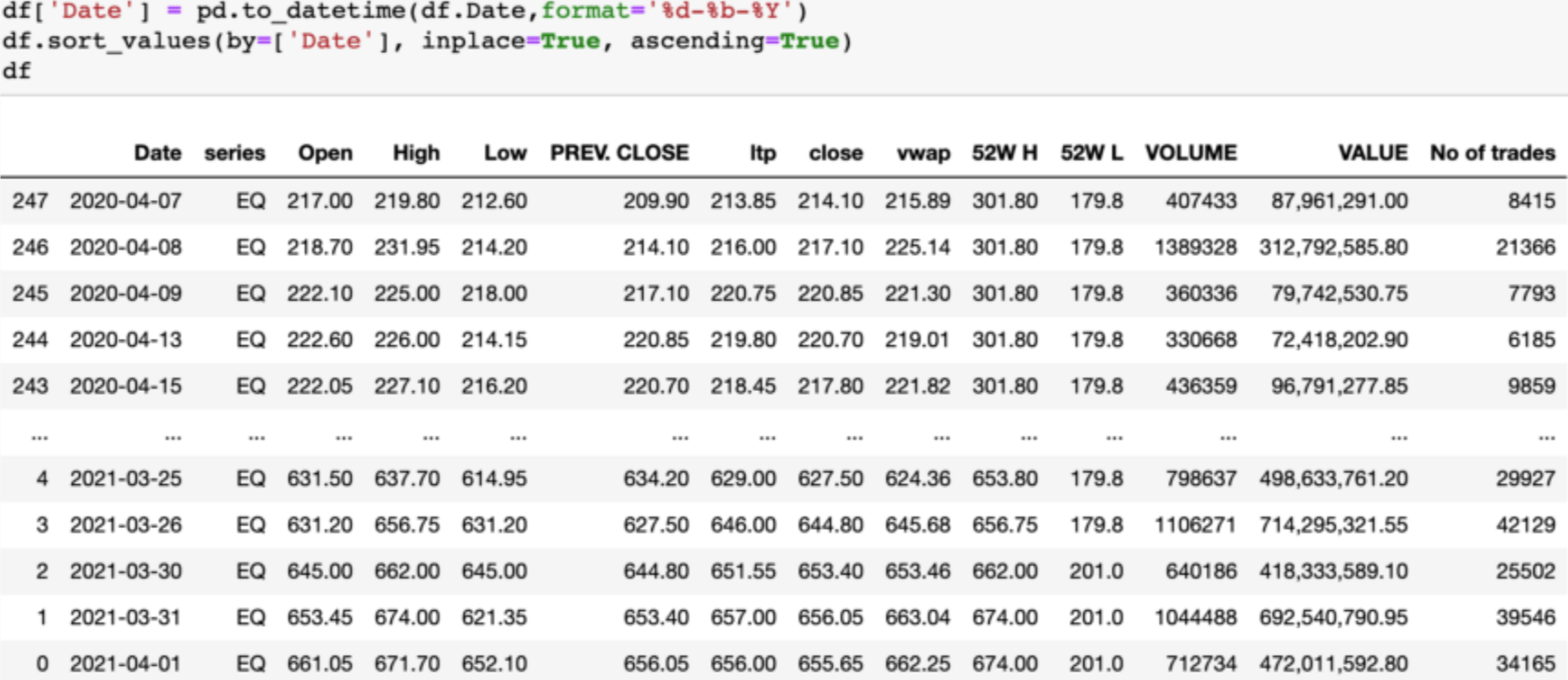``````from matplotlib import pyplot as plt
import numpy as np
x = df[‘Date’]
y = df[‘ltp’]
plt.plot(x, y)
``````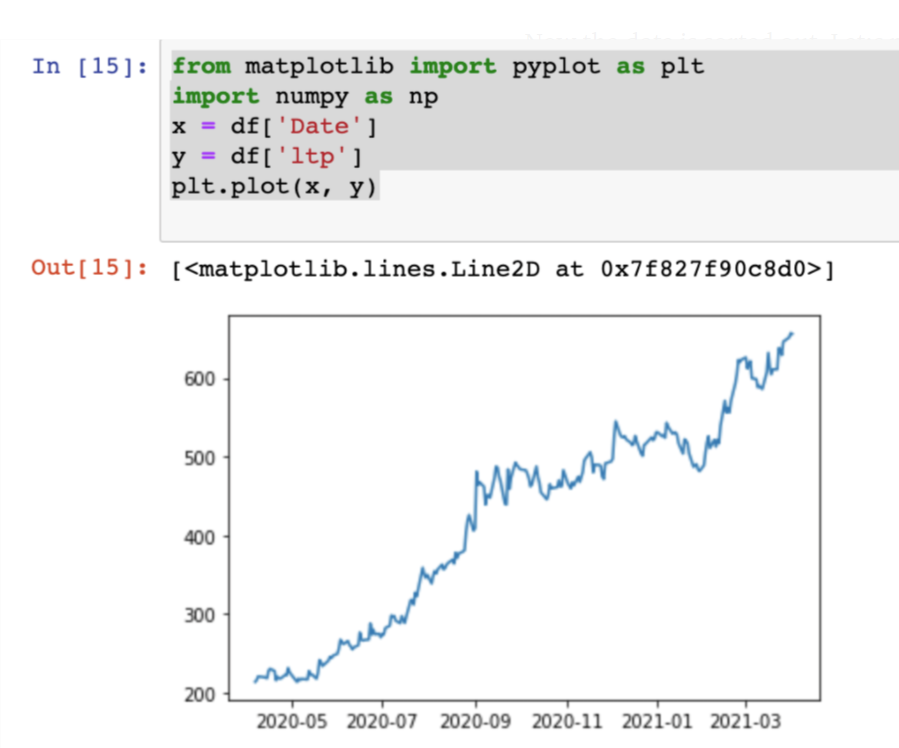``````dfdata = df[[“Date”,”ltp”]]
dfdata.rename(columns={“Date”:”ds”}, inplace=True)
dfdata.rename(columns={“ltp”:”y”}, inplace=True)
``````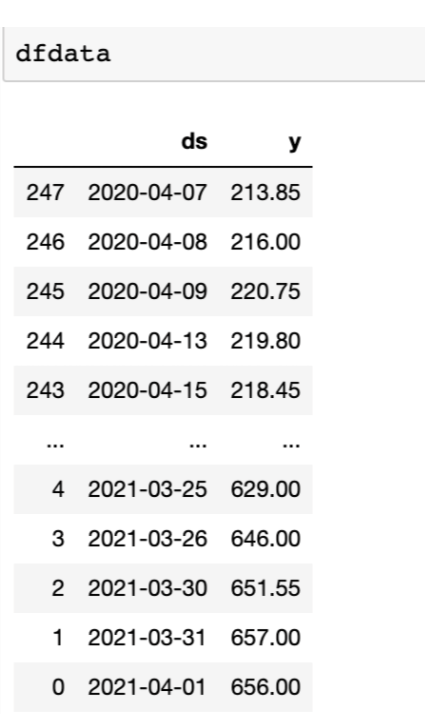``````from fbprophet import Prophet
m = Prophet()
m.fit(dfdata)
``````

``````future = m.make_future_dataframe(periods=300)
future.tail()
``````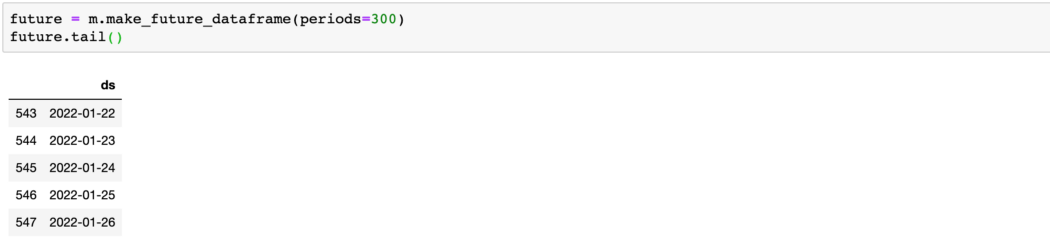``````forecast = m.predict(future)
dfforecast= forecast[[“ds”, “yhat”, “yhat_lower”, “yhat_upper”]]
pd.set_option(‘display.max_rows’, dfforecast.shape+1)
dfforecast
``````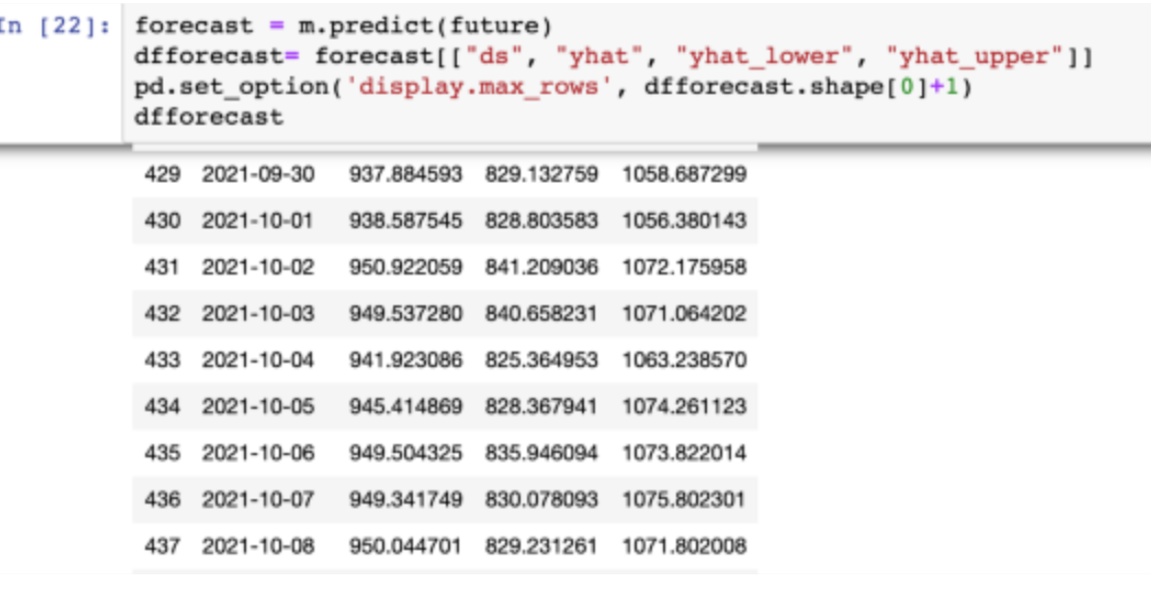``````fig1 = m.plot(forecast)
``````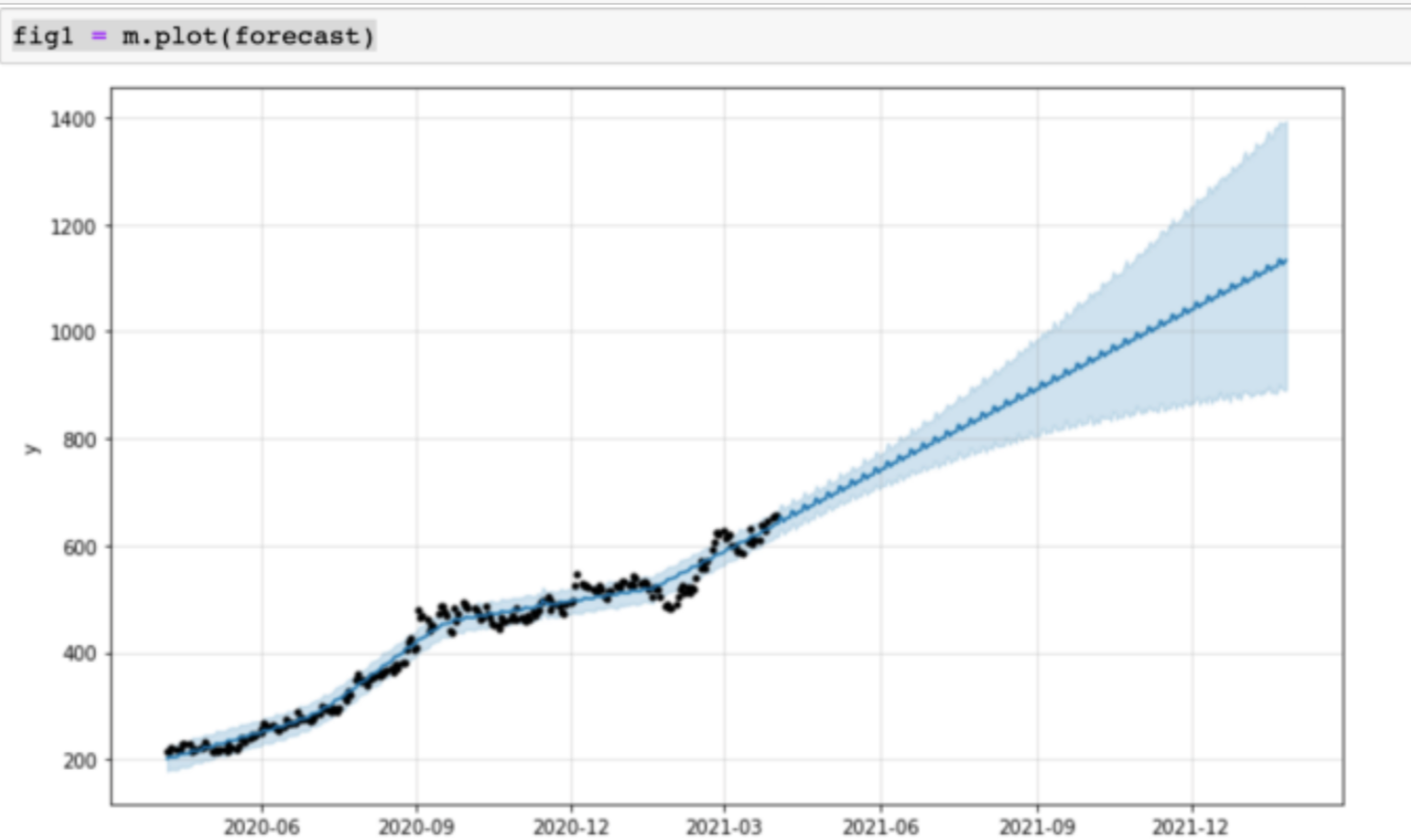``````fig2 = m.plot_components（预测）
``````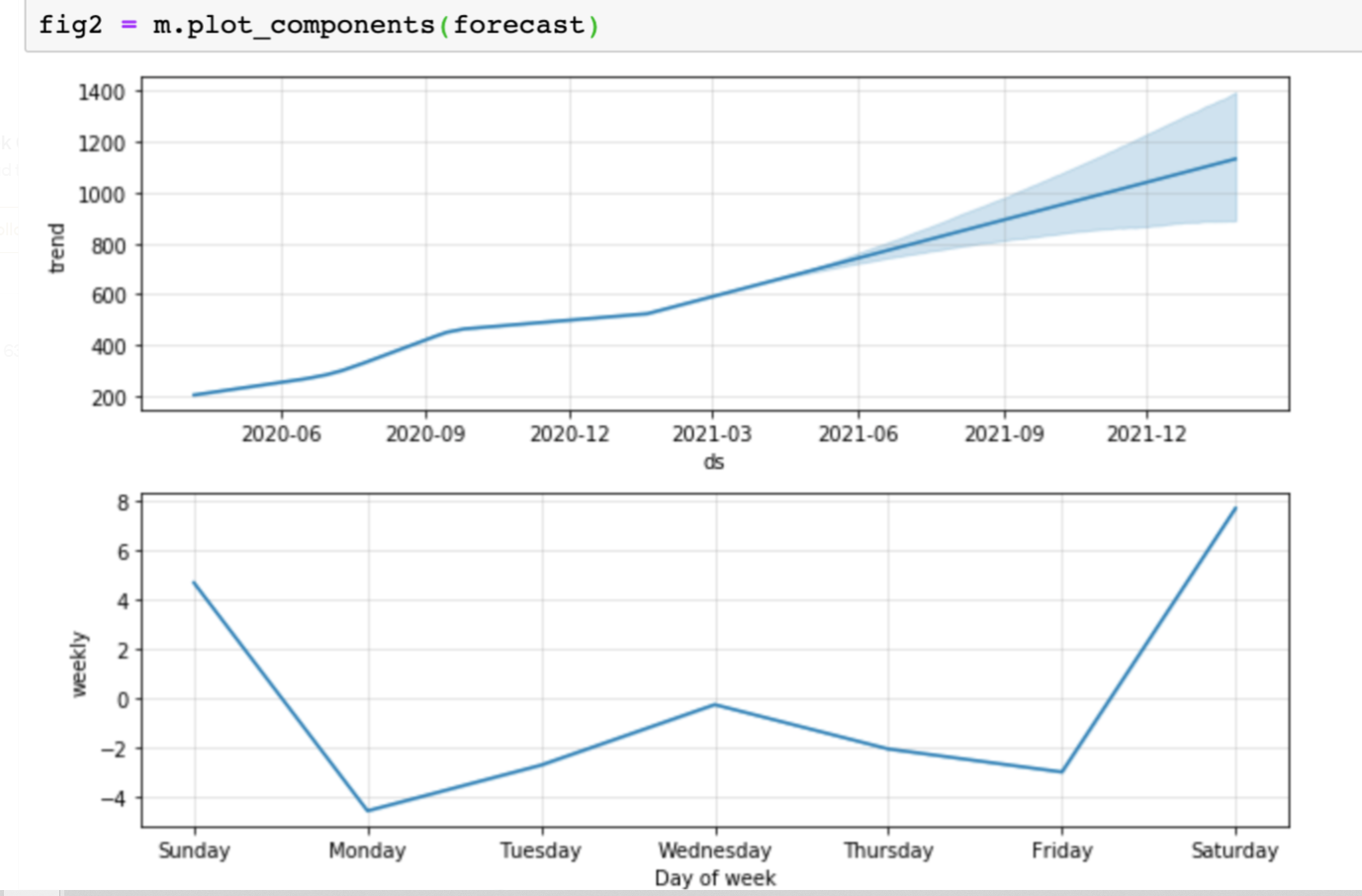``````from fbprophet.plot import plot_plotly
import plotly.offline as py
py.init_notebook_mode()
fig = plot_plotly(m, forecast) # This returns a plotly Figure
py.iplot(fig)
``````